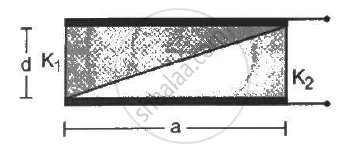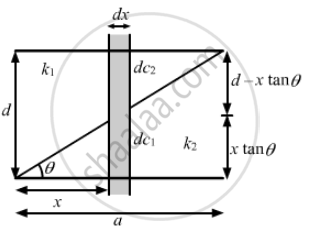Department of Pre-University Education, KarnatakaPUC Karnataka Science Class 12
Share

# A Capacitor is Formed by Two Square Metal-plates of Edge A, Separated by a Distance D. Dielectrics of Dielectric Constant K1 and K2 Are Filled in the Gap as Shown in Figure . Find the Capacitance. - Physics

ConceptCapacitors and Capacitance

#### Question

A capacitor is formed by two square metal-plates of edge a, separated by a distance d. Dielectrics of dielectric constant K1 and K2 are filled in the gap as shown in figure . Find the capacitance.#### SolutionLet us consider an elemental capacitor of width dx at a distance x from the left end of the capacitor. It has two capacitive elements of dielectric constants K1 and K2 with plate separations (x tan θ) and (d − x tan θ) in series, respectively. The areas of the plates of the capacitors are adx.

The capacitances of the capacitive elements of the elemental capacitor are :

dC_1 = (∈_0K_2(adx))/(x tan θ) , dC_2 = (∈_0K_1(adx))/(d - x tan θ)

The net capacitance of the elemental capacitor is given by

1/(dC)= 1/(dC_1) + 1/(dC_2)

1/(dC) = (x tanθ)/(∈_0K_2(adx)) + (d-x tanθ)/(∈_0K_1(adx))

⇒ dC = (∈_0K_1K_2(adx))/(K_1 x tanθ + K_2(d-x tanθ)

Thus, integrating the above expression to calculate the net capacitance

C = ∫_0^a dC = ∫_0^a (∈_0K_1K_2adx)/(K_1x tanθ+ K_2(d-x tanθ)

⇒ C = ∈_0K_1K_2a ∫_0^a (dx)/(K_2d + x tanθ(K_1 - K_2)

⇒ C = (∈_0K_1K_2a)/(tanθ(K_1 - K_2))[log_e[K_2d + x tanθ(K_1 - K_2) ]]_0^a

⇒ C = (∈_0K_1K_2a)/(tan θ(K_1 - K_2)) [ log_e[K_2d + a tan θ(K_1 - K_2)] - log_e K_2d]

As we know that tan  θ = d/a substituting in the expression for capacitance C.
Now,

⇒ C = (∈_0K_1K_2a)/(d/a xx (K_1 - K_2) )[ log_e[K_2d + a xx d/a(K_1 - K_2)] - log_e K_2d]

⇒ C = (∈_0K_1K_2a)/(d/a xx (K_1 - K_2) )[ log_e K_1d - log_e K_2d ]

⇒ C = (∈_0K_1K_2a^2)/(d(K_1 - K_2)) [log_e (K_1/K_2)]

Is there an error in this question or solution?

#### Video TutorialsVIEW ALL 

Solution A Capacitor is Formed by Two Square Metal-plates of Edge A, Separated by a Distance D. Dielectrics of Dielectric Constant K1 and K2 Are Filled in the Gap as Shown in Figure . Find the Capacitance. Concept: Capacitors and Capacitance.
S